This function determines the critical values for isolating a central portion of a distribution with a specified probability. This is designed to work especially well for symmetric distributions, but it can be used with any distribution.

cdist(
dist = "norm",
p,
plot = TRUE,
verbose = FALSE,
invisible = FALSE,
digits = 3L,
xlim,
ylim,
resolution = 500L,
return = c("values", "plot"),
pattern = c("rings", "stripes"),
...,
refinements = list()
)

xcgamma(
p,
shape,
rate = 1,
scale = 1/rate,
lower.tail = TRUE,
log.p = FALSE,
...
)

xct(p, df, ncp, lower.tail = TRUE, log.p = FALSE, ...)

xcchisq(p, df, ncp = 0, lower.tail = TRUE, log.p = FALSE, ...)

xcf(p, df1, df2, lower.tail = TRUE, log.p = FALSE, ...)

xcbinom(p, size, prob, lower.tail = TRUE, log.p = FALSE, ...)

xcpois(p, lambda, lower.tail = TRUE, log.p = FALSE, ...)

xcgeom(p, prob, lower.tail = TRUE, log.p = FALSE, ...)

xcnbinom(p, size, prob, mu, lower.tail = TRUE, log.p = FALSE, ...)

xcbeta(p, shape1, shape2, ncp = 0, lower.tail = TRUE, log.p = FALSE, ...)

## Arguments

dist

a character string naming a distribution family (e.g., "norm"). This will work for any family for which the usual d/p/q functions exist.

p

the proportion to be in the central region, with equal proportions in either "tail".

plot

a logical indicating whether a plot should be created

verbose

a logical indicating whether a more verbose output value should be returned.

invisible

a logical

digits

the number of digits desired

xlim

x limits. By default, these are chosen to show the central 99.8\ of the distribution.

ylim

y limits

resolution

number of points used for detecting discreteness and generating plots. The default value of 5000 should work well except for discrete distributions that have many distinct values, especially if these values are not evenly spaced.

return

If "plot", return a plot. If "values", return a vector of numerical values.

pattern

One of "stripes" or "rings". In the latter case, pairs of regions (from the outside to the inside) are grouped together for coloring and probability calculation.

...

additional arguments passed to the distribution functions. Typically these specify the parameters of the particular distribution desired. See the examples.

refinements

A list of refinements to the plot. See ggformula::gf_refine().

shape

shape and scale parameters. Must be positive, scale strictly.

rate

an alternative way to specify the scale.

scale

shape and scale parameters. Must be positive, scale strictly.

lower.tail

logical; if TRUE (default), probabilities are $$P[X \le x]$$, otherwise, $$P[X > x]$$.

log.p

logical; if TRUE, probabilities/densities $$p$$ are returned as $$log(p)$$.

df

degrees of freedom ($$> 0$$, maybe non-integer). df = Inf is allowed.

ncp

non-centrality parameter $$\delta$$; currently except for rt(), only for abs(ncp) <= 37.62. If omitted, use the central t distribution.

df1

degrees of freedom. Inf is allowed.

df2

degrees of freedom. Inf is allowed.

size

number of trials (zero or more).

prob

probability of success on each trial.

lambda

vector of (non-negative) means.

mu

alternative parametrization via mean: see ‘Details’.

shape1

non-negative parameters of the Beta distribution.

shape2

non-negative parameters of the Beta distribution.

## Value

a pair of numbers indicating the upper and lower bounds, unless verbose is TRUE, in which case a 1-row data frame is returned containing these bounds, the central probability, the tail probabilities, and the name of the distribution.

## Note

This function is still experimental and changes the input or output formats are possible in future versions of the package.

## Examples

cdist( "norm", .95)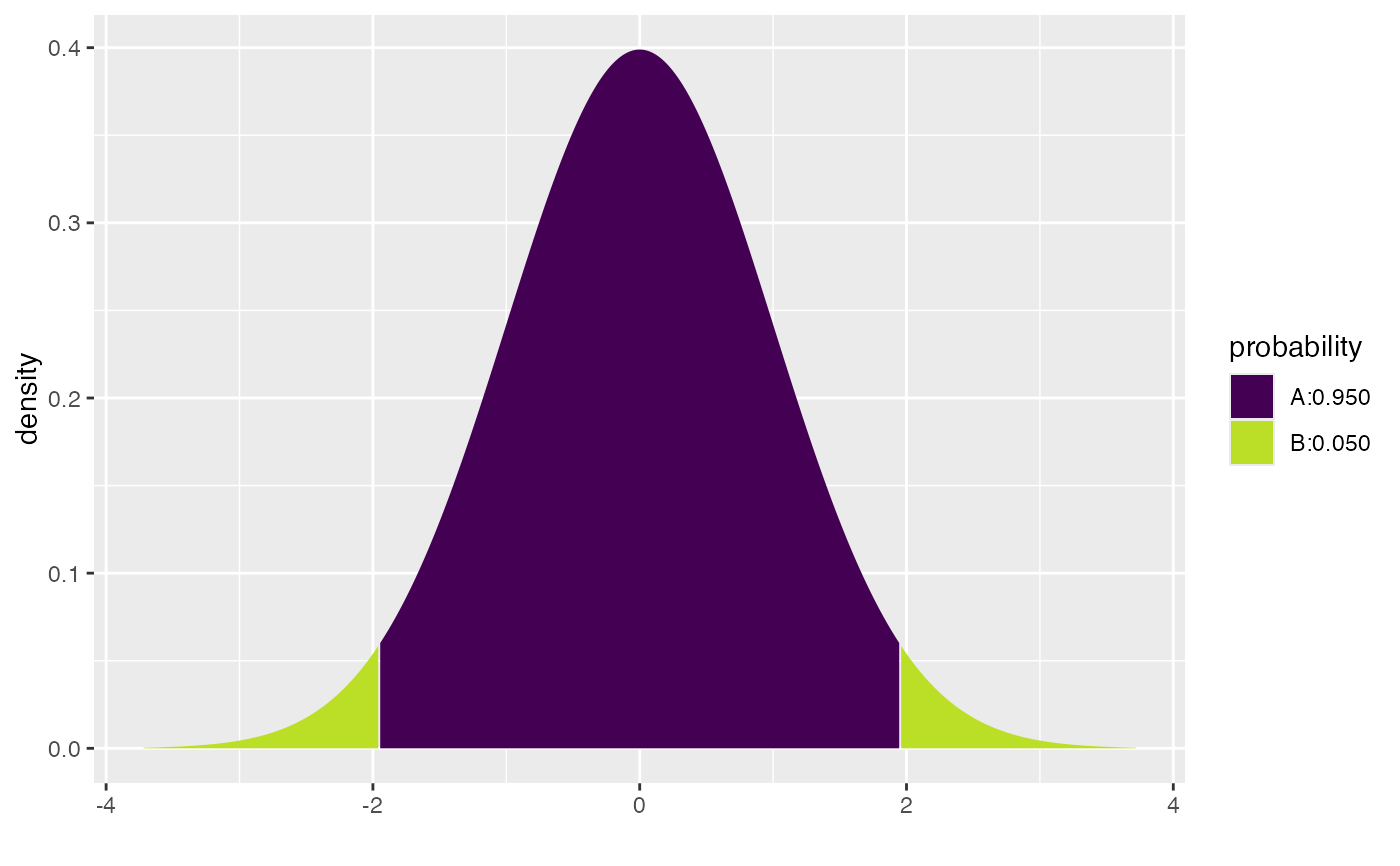#>  -1.959964  1.959964
cdist( "t", c(.90, .95, .99), df=5)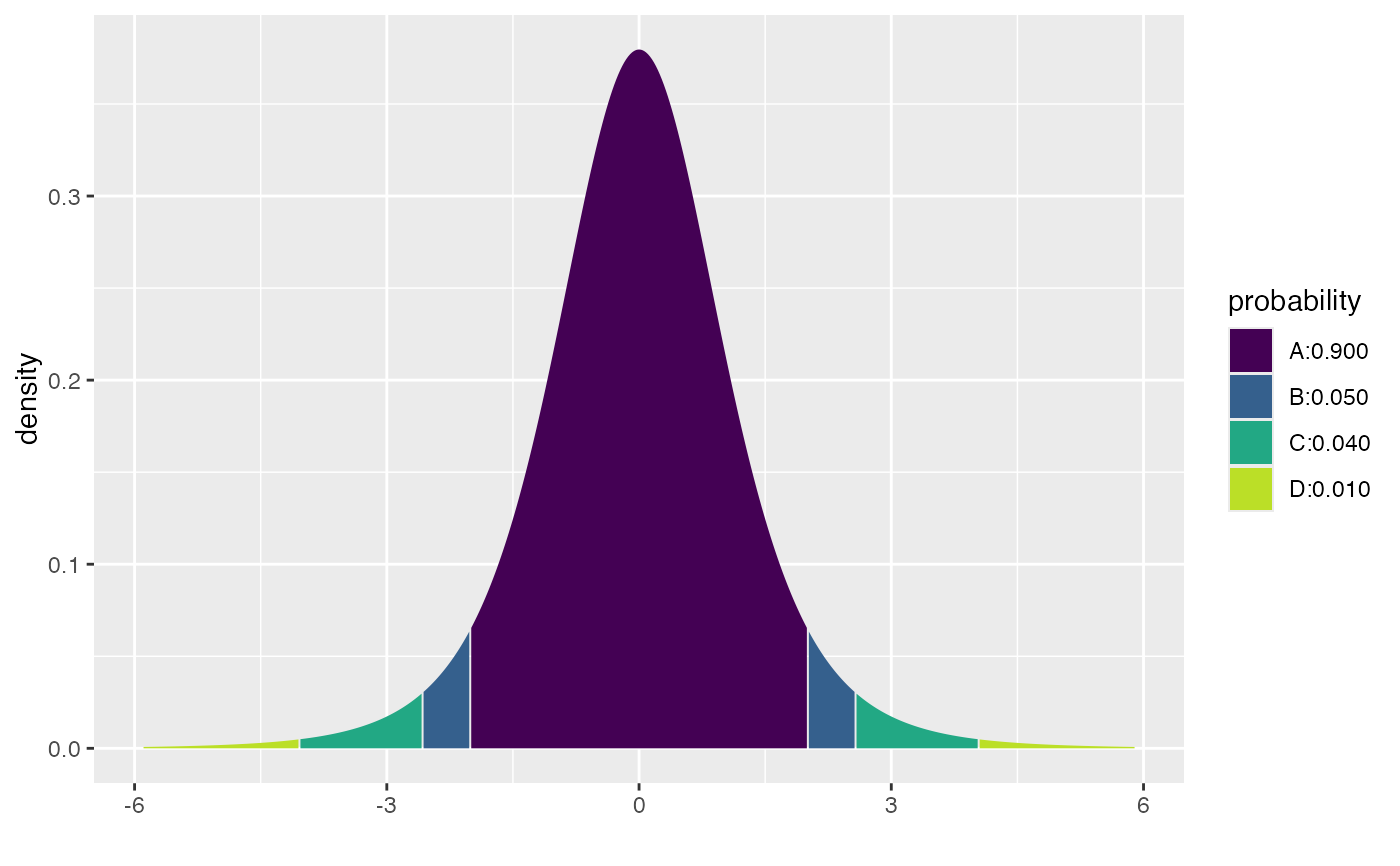#>  -4.032143 -2.570582 -2.015048  2.015048  2.570582  4.032143
cdist( "t", c(.90, .95, .99), df=50)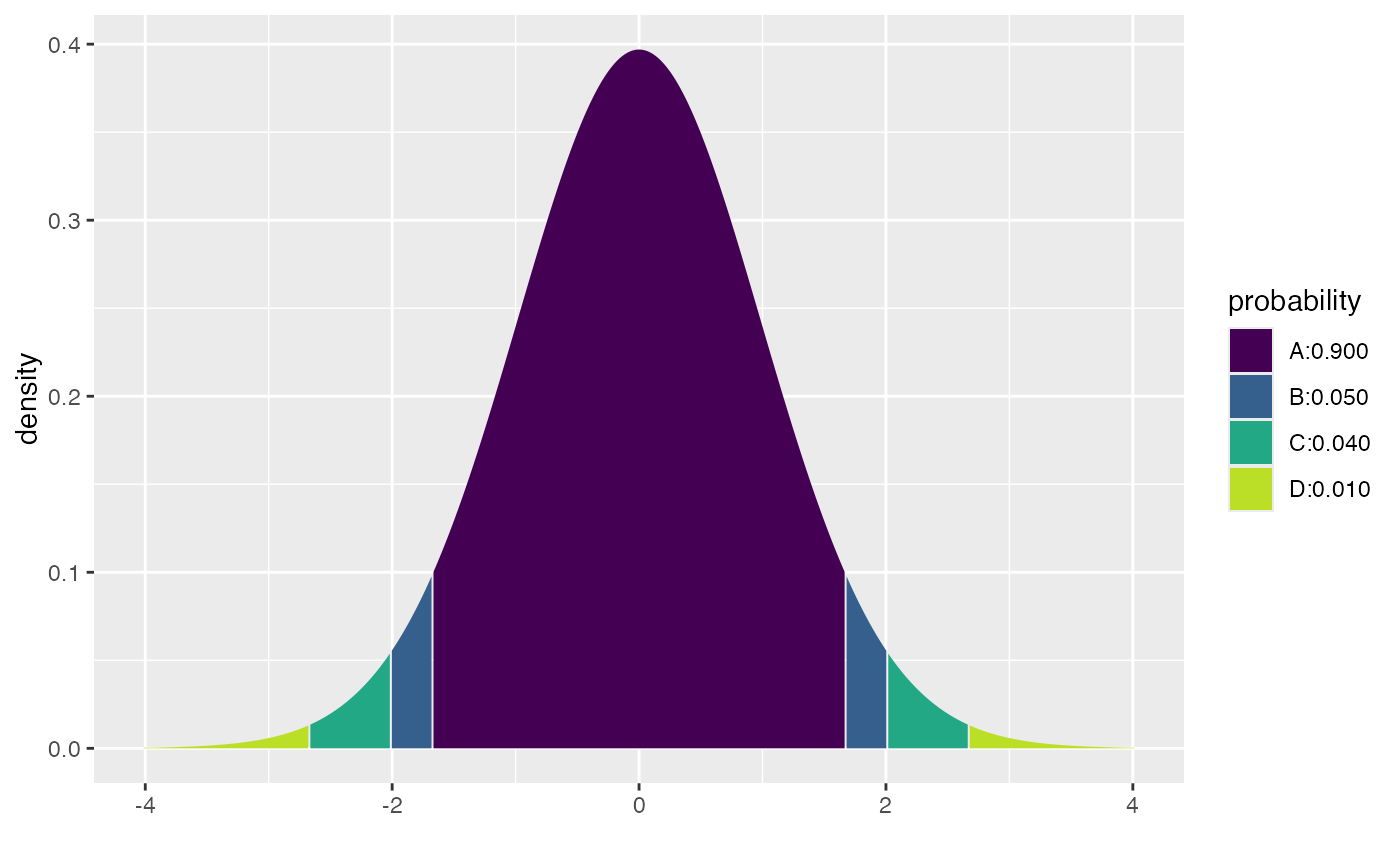#>  -2.677793 -2.008559 -1.675905  1.675905  2.008559  2.677793
# plotting doesn't work well when the parameters are not constant
cdist( "t", .95, df=c(3,5,10,20), plot = FALSE)
#> Error in stop_bad_type(x, expected, actual = actual, what = what, arg = arg,     recycle = recycle, message = message, .subclass = c(.subclass,         "purrr_error_bad_vector")): Result 1 must be a single double, not a double vector of length 4
cdist( "norm", .95, mean=500, sd=100 )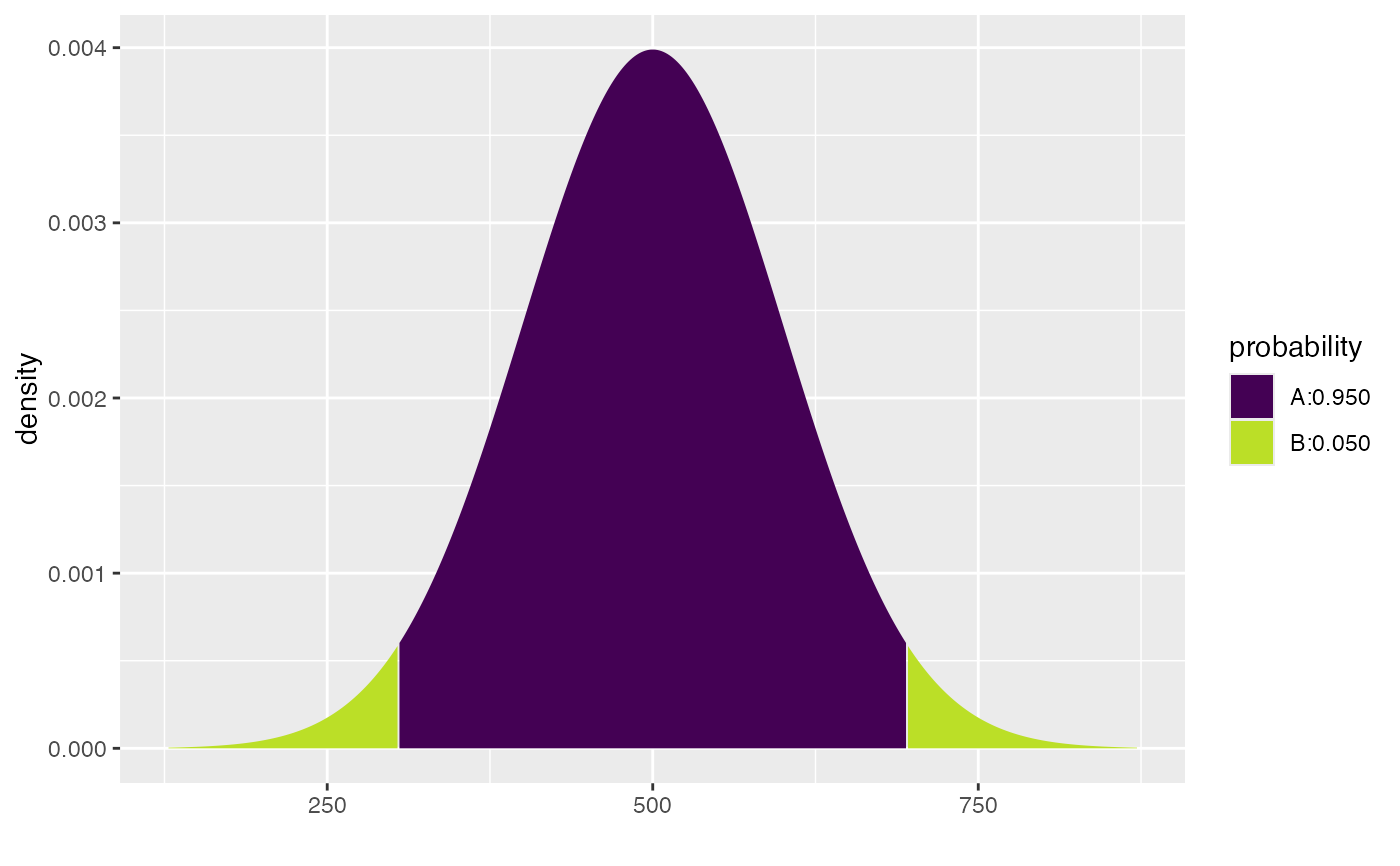#>  304.0036 695.9964
cdist( "chisq", c(.90, .95), df=3 )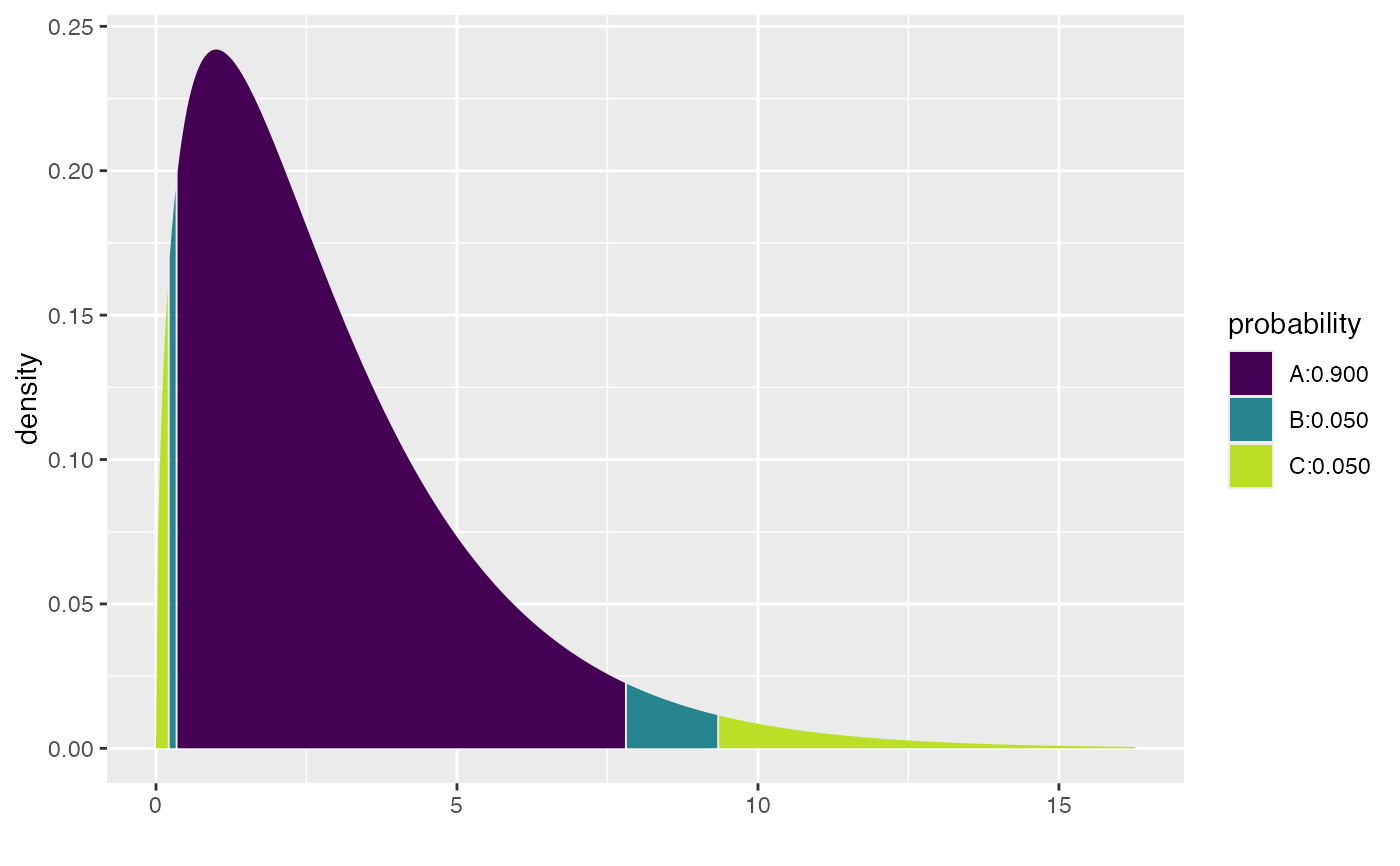#>  0.2157953 0.3518463 7.8147279 9.3484036
# CI
x <- rnorm(23, mean = 10, sd = 2)
cdist("t", p = 0.95, df=22)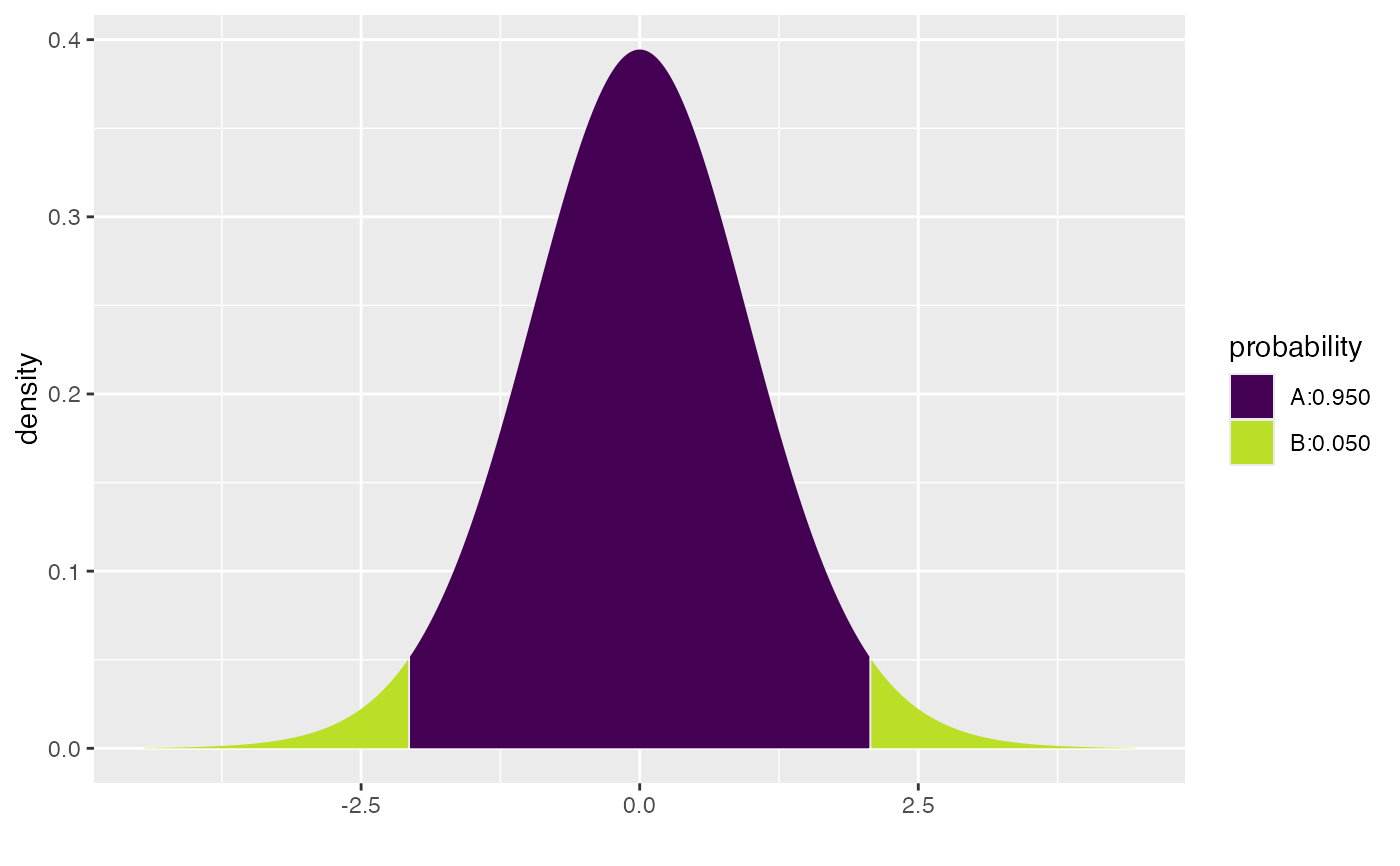#>  -2.073873  2.073873
mean(x) + cdist("t", p = 0.95, df=22) * sd(x) / sqrt(23)#>   8.817601 10.393075
confint(t.test(x))
#>   mean of x    lower    upper level
#> 1  9.605338 8.817601 10.39308  0.95
cdist("t", p = 0.95, df=22, verbose = TRUE)
#> Verbose output not yet implemented.#>  -2.073873  2.073873